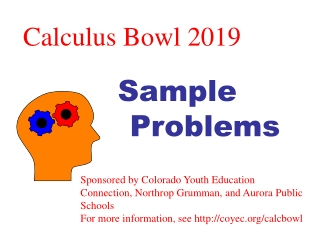# Calculus Bowl 2019 - PowerPoint PPT PresentationDownload PresentationCalculus Bowl 2019

Calculus Bowl 2019Download Presentation## Calculus Bowl 2019

- - - - - - - - - - - - - - - - - - - - - - - - - - - E N D - - - - - - - - - - - - - - - - - - - - - - - - - - -
##### Presentation Transcript

1. Calculus Bowl 2019 Sample Problems Sponsored by Colorado Youth Education Connection, Northrop Grumman, and Aurora Public Schools For more information, see http://coyec.org/calcbowl

2. Problem 1

3. Problem 2

4. Problem 3

5. Problem 4

6. Problem 5

7. Problem 6

8. Problem 7

9. Problem 8

10. Problem 9

11. Problem 10 P(x) (2,2) (-1, -1)

12. Problem 11

13. Problem 12

14. Problem 13 y y=p(x)

15. Problem 14 g(x) (2,1)

16. Problem 15

17. Problem 16

18. Problem 17

19. Problem 18

20. Problem 19

21. Problem 20

22. Problem 21

23. Problem 22 y x

24. Problem 23

25. Problem 25

26. Problem 26

27. Problem 28

28. Problem 29

29. Problem 31